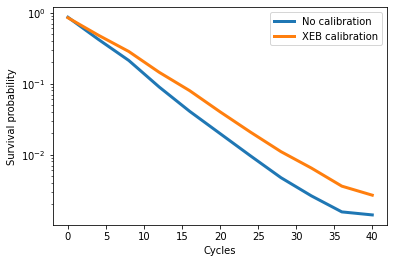# XEB calibration: Example and benchmark

This tutorial shows a detailed example and benchmark of XEB calibration, a calibration technique introduced in the Calibration: Overview and API tutorial.

Disclaimer: The data shown in this tutorial is exemplary and not representative of the QCS in production.

## Setup

try:
import cirq
except ImportError:
!pip install --quiet cirq --pre


import cirq
from cirq.experiments import random_quantum_circuit_generation as rqcg

import matplotlib.pyplot as plt
import numpy as np
import tqdm


## Select qubits

First we select a processor and calibration metric(s) to visualize the latest calibration report.Using this report as a guide, we select a good set of qubits.

# Select qubit indices here.
qubit_indices = [
(2, 5), (2, 6), (2, 7), (2, 8), (3, 8),
(3, 7), (3, 6), (3, 5), (4, 5), (4, 6)
]
qubits = [cirq.GridQubit(*idx) for idx in qubit_indices]


An example random circuit on these qubits (used as the forward operations of the Loschmidt echo) is shown below.

create_random_circuit(qubits, cycles=10, seed=1)


## Set up XEB calibration

Now we specify the cycle depths and other options for XEB calibration below. Note that all cirq.FSimGate parameters are characterized by default.

xeb_options = cg.LocalXEBPhasedFSimCalibrationOptions(
cycle_depths=(5, 25, 50, 100),
n_processes=1,
fsim_options=cirq.experiments.XEBPhasedFSimCharacterizationOptions(
characterize_theta=False,
characterize_zeta=True,
characterize_chi=True,
characterize_gamma=True,
characterize_phi=False,
),
)


## Run a Loschmidt echo benchmark

"""Setup the Loschmidt echo experiment."""
cycle_values = range(0, 40 + 1, 4)
nreps = 20_000
trials = 10

sampler = cg.get_engine_sampler(
project_id=project_id,
processor_id=processor_id,
gate_set_name="sqrt_iswap",
)

loschmidt_echo_batch = [
create_loschmidt_echo_circuit(qubits, cycles=c, seed=trial)
for trial in range(trials) for c in cycle_values
]


### Without calibration

First we run the Loschmidt echo without calibration.

# Run on the engine.
raw_results = sampler.run_batch(programs=loschmidt_echo_batch, repetitions=nreps)

# Convert measurements to survival probabilities.
raw_probs = np.array(
[to_ground_state_prob(*res) for res in raw_results]
).reshape(trials, len(cycle_values))


### With XEB calibration

Now we perform XEB calibration.

# Get characterization requests.
characterization_requests = cg.prepare_characterization_for_operations(loschmidt_echo_batch, xeb_options)

# Characterize the requests on the engine.
characterizations = cg.run_calibrations(characterization_requests, sampler)

# Make compensations to circuits in the Loschmidt echo batch.
xeb_calibrated_batch = [
cg.make_zeta_chi_gamma_compensation_for_moments(circuit, characterizations).circuit
for circuit in loschmidt_echo_batch
]

100%|██████████| 45/45 [00:46<00:00,  1.04s/it]
100%|██████████| 45/45 [01:50<00:00,  2.45s/it]
100%|██████████| 45/45 [01:00<00:00,  1.34s/it]
100%|██████████| 45/45 [02:24<00:00,  3.20s/it]


And run the XEB calibrated batch below.

# Run on the engine.
xeb_results = sampler.run_batch(programs=xeb_calibrated_batch, repetitions=nreps)

# Convert measurements to survival probabilities.
xeb_probs = np.array(
[to_ground_state_prob(*res) for res in xeb_results]
).reshape(trials, len(cycle_values))


### Compare results

The next cell plots the results.

plt.semilogy(cycle_values, np.average(raw_probs, axis=0), lw=3, label="No calibration")
plt.semilogy(cycle_values, np.average(xeb_probs, axis=0), lw=3, label="XEB calibration")

plt.xlabel("Cycles")
plt.ylabel("Survival probability")
plt.legend();A smaller (in magnitude) slope indicates lower two-qubit gate errors. You should see that XEB calibration produces lower errors than no calibration in the Loschmidt echo benchmark.

[{ "type": "thumb-down", "id": "missingTheInformationINeed", "label":"Missing the information I need" },{ "type": "thumb-down", "id": "tooComplicatedTooManySteps", "label":"Too complicated / too many steps" },{ "type": "thumb-down", "id": "outOfDate", "label":"Out of date" },{ "type": "thumb-down", "id": "samplesCodeIssue", "label":"Samples / code issue" },{ "type": "thumb-down", "id": "otherDown", "label":"Other" }]
[{ "type": "thumb-up", "id": "easyToUnderstand", "label":"Easy to understand" },{ "type": "thumb-up", "id": "solvedMyProblem", "label":"Solved my problem" },{ "type": "thumb-up", "id": "otherUp", "label":"Other" }]